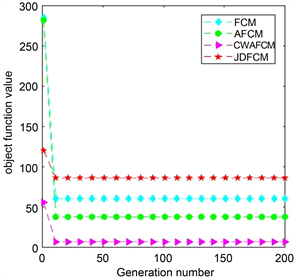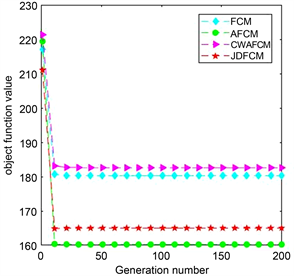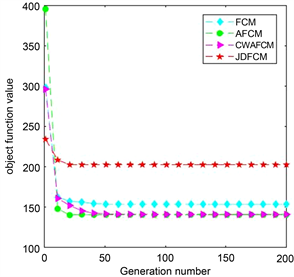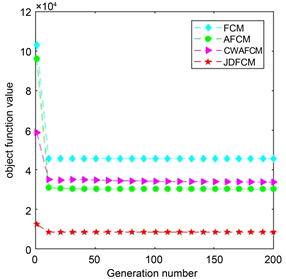# 基于PSO-TVAC的中心自适应权的FCM聚类算法FCM Clustering Algorithm Based on PSO-TVAC Algorithm with Adaptively Weighted Centers

DOI: 10.12677/AAM.2021.104104, PDF, HTML, XML, 下载: 19  浏览: 814  国家自然科学基金支持

Abstract: The traditional FCM algorithm relies on the initial clustering center, is sensitive to noise, is easy to fall into local optimum, and tends to classify most classes. In this paper, a FCM clustering algorithm based on PSO-TVAC algorithm with adaptively weighted centers is proposed. The new algorithm introduces the weight vector φ of centers and the adaptive exponent q into the objective function to distinguish the different importance of each cluster center. The exponent q and fuzzy factor m are optimized by particle swarm optimization (PSO-TVAC). Secondly, CWAFCM is combined with synthetic minority oversampling technique (SMOTE) to cluster unbalanced data. The results of experiments on six datasets (four balanced datasets and two unbalanced datasets) show that CWAFCM algorithm can effectively optimize the clustering effect and improve the clustering accuracy on unbalanced dataset.

1. 引言

FCM算法是一种经典的聚类方法，由Dunn  在1973年提出。由于其简单易实现，并能获得较好的结果而广泛应用于数据挖掘、模式识别、信号处理、图像分割等领域中   。但FCM算法仍然存在依赖初始聚类中心、对噪声敏感度、容易陷入局部最优等缺陷。近些年来，许多改进的FCM算法    和聚类有效性指标被提出以提高聚类的效果。如Ayan Seal等人  提出一种基于Jeffreys散度为相似度度量的改进FCM算法(JDFCM)，大大提高了聚类性能。Niteesh Kumar等人  给出两种不同的距离度量方法来适应噪声环境，提出了AMFCM算法和EMFCM算法。同时，FCM聚类算法与其他优化算法的结合也大大提高了算法的性能。例如，文献  讨论了一种基于FCM和FPSO的混合聚类算法(FCMPSO)用来提高聚类效果。因为在噪声和样本分布不均衡的环境下，聚类结果不理想，文献  考虑到每个样本的重要性，提出了具有样本自适应权的FCM算法(AFCM)。

2. 传统的FCM算法与SMOTE技术

2.1. 模糊c均值算法(FCM)

FCM算法主要通过迭代方式最小化其目标函数以获得样本集的模糊划分，它将靠近聚类中心的样本点赋予较高的隶属度，而远离聚类中心的样本点赋予较低的隶属度。设 $X=\left\{{x}_{1},{x}_{2},\cdots ,{x}_{n}\right\}$ 是数据集，n是样本数，c是已知的类别数。传统的FCM算法以欧氏距离作为相似度度量，其目标函数为：

$J\left(U,V\right)=\underset{i=1}{\overset{n}{\sum }}\underset{j=1}{\overset{c}{\sum }}{\mu }_{ji}^{m}{‖{x}_{i}-{v}_{j}‖}^{2}$ (1)

${\mu }_{ji}\in \left[0,1\right],\text{ }\underset{j=1}{\overset{c}{\sum }}{\mu }_{ji}=1,\text{\hspace{0.17em}}i=1,2\cdots ,n$ (2)

$U={\left({\mu }_{ji}\right)}_{c×n}$ 表示模糊隶属度矩阵， $V=\left\{{v}_{1},{v}_{2},\cdots ,{v}_{c}\right\}$ 是所有聚类中心的集合。FCM算法通过迭代更新隶属度矩阵与聚类中心以实现聚类的目的，其迭代公式为

${\mu }_{ji}=\frac{1}{\underset{k=1}{\overset{c}{\sum }}{\left(\frac{‖{x}_{i}-{v}_{j}‖}{‖{x}_{i}-{v}_{k}‖}\right)}^{\frac{2}{m-1}}}$ (3)

${v}_{j}=\frac{\underset{i=1}{\overset{n}{\sum }}{\mu }_{ji}^{m}{x}_{i}}{\underset{i=1}{\overset{n}{\sum }}{\mu }_{ji}^{m}}$ (4)

$J=1\cdot \underset{j=1}{\overset{c}{\sum }}{\mu }_{j1}^{m}{‖{x}_{1}-{v}_{j}‖}^{2}+1\cdot \underset{j=1}{\overset{c}{\sum }}{\mu }_{j2}^{m}{‖{x}_{2}-{v}_{j}‖}^{2}+\cdots +1\cdot \underset{j=1}{\overset{c}{\sum }}{\mu }_{jn}^{m}{‖{x}_{n}-{v}_{j}‖}^{2}$ (5)

${G}_{a}\left(\psi ,U,V\right)=\underset{i=1}{\overset{n}{\sum }}{\psi }_{i}^{p}\underset{j=1}{\overset{c}{\sum }}{\mu }_{ji}^{m}{‖{x}_{i}-{v}_{j}‖}^{2}$ (6)

$\underset{j=1}{\overset{c}{\sum }}{\mu }_{ji}=1,\text{ }\underset{i=1}{\overset{n}{\prod }}{\psi }_{i}=1$ (7)

${\psi }_{i}={\left\{\frac{{\left[\underset{i=1}{\overset{n}{\prod }}\left(\underset{j=1}{\overset{c}{\sum }}{\mu }_{ji}^{m}{‖{x}_{i}-{v}_{j}‖}^{2}\right)\right]}^{\frac{1}{n}}}{\underset{j=1}{\overset{c}{\sum }}{\mu }_{ji}^{m}{‖{x}_{i}-{v}_{j}‖}^{2}}\right\}}^{\frac{1}{p}}$ (8)

${v}_{j}=\frac{\underset{i=1}{\overset{n}{\sum }}{\psi }_{i}{\mu }_{ji}^{m}{x}_{i}}{\underset{i=1}{\overset{n}{\sum }}{\psi }_{i}{\mu }_{ji}^{m}}$ (9)

AFCM算法有效减少了噪声点的干扰，也适应于样本分布不均衡的情况。

2.2. SMOTE技术

1) 在少类样本中选取样本 ${x}_{i}$，计算 ${x}_{i}$ 到每个少类样本的欧氏距离，选取K个距离最小的样本作为K近邻。

2) 根据多类样本与少类样本之间的不平衡比率确定采样倍率N，对于少类中的每个样本 ${x}_{i}$，从其K近邻中选取若干个样本，不妨设为 ${x}_{n}$

3) 对于每个随机选择出的 ${x}_{n}$ 与原样本 ${x}_{i}$，按照式(10)，合成的新样本 ${x}_{new}$

${x}_{new}={x}_{i}+rand\left(0,1\right)\left({x}_{i}-{x}_{n}\right)$ (10)

3. 基于PSO-TVAC的中心自适应权的FCM算法

AFCM  算法启示我们，在聚类过程中将每个样本区别以待可以减少噪声干扰，提高算法性能。同样，FCM算法中，聚类中心起着非常重要的作用，它决定了最终的聚类结果，但其结果依赖于初始中心的选取。为减少这种依赖性，本文将强调每个中心的重要性，提出一种基于PSO-TVAC的中心自适应权的FCM聚类算法(CWAFCM)。

3.1. 中心自适应权的FCM模型

$J=1\cdot \underset{j=1}{\overset{c}{\sum }}{\mu }_{j1}^{m}{‖{x}_{1}-{v}_{j}‖}^{2}+1\cdot \underset{j=1}{\overset{c}{\sum }}{\mu }_{j2}^{m}{‖{x}_{2}-{v}_{j}‖}^{2}+\cdots +1\cdot \underset{j=1}{\overset{c}{\sum }}{\mu }_{jn}^{m}{‖{x}_{n}-{v}_{j}‖}^{2}$, (11)

${G}_{c}\left(\phi ,U,V\right)=\underset{j=1}{\overset{c}{\sum }}{\phi }_{j}^{q}\underset{i=1}{\overset{n}{\sum }}{\mu }_{ji}^{m}{‖{x}_{i}-{v}_{j}‖}^{2}$ (12)

$L\left(\phi ,U,V\right)=\left(\underset{j=1}{\overset{c}{\sum }}{\phi }_{j}^{q}\underset{i=1}{\overset{n}{\sum }}{\mu }_{ji}^{m}{‖{x}_{i}-{v}_{j}‖}^{2}\right)+\beta \left(\underset{j=1}{\overset{c}{\prod }}{\phi }_{j}-1\right)+\underset{i=1}{\overset{n}{\sum }}{\lambda }_{i}\left(\underset{j=1}{\overset{c}{\sum }}{\mu }_{ji}-1\right)$

$L\left(\phi ,U,V\right)$ 中对 $U,\phi ,V$ 求偏导并令其等于0有

$\frac{\partial L}{\partial {\mu }_{ji}}=m{\phi }_{j}^{q}{\mu }_{ji}^{m-1}{‖{x}_{i}-{v}_{j}‖}^{2}+{\lambda }_{i}=0$

$\frac{\partial L}{\partial {\phi }_{j}}=q{\phi }_{j}^{q-1}\underset{i=1}{\overset{n}{\sum }}{\mu }_{ji}^{m}{‖{x}_{i}-{v}_{j}‖}^{2}+\beta \underset{l=1,l\ne j}{\overset{c}{\prod }}{\phi }_{l}=0$

$\frac{\partial L}{\partial {v}_{j}}=-\underset{j=1}{\overset{c}{\sum }}{\phi }_{j}^{q}{\mu }_{ji}^{m}\left({x}_{i}-{v}_{j}\right)=0$

${\mu }_{ji}=\frac{1}{\underset{k=1}{\overset{c}{\sum }}{\left[\frac{{\phi }_{j}{‖{x}_{i}-{v}_{j}‖}^{2}}{{\phi }_{k}{‖{x}_{i}-{v}_{k}‖}^{2}}\right]}^{\frac{1}{m-1}}}$ (13)

${\phi }_{j}={\left\{\frac{{\left[\underset{j=1}{\overset{c}{\prod }}\left(\underset{i=1}{\overset{n}{\sum }}{\mu }_{ji}^{m}{‖{x}_{i}-{v}_{j}‖}^{2}\right)\right]}^{\frac{1}{c}}}{\underset{i=1}{\overset{n}{\sum }}{\mu }_{ji}^{m}{‖{x}_{i}-{v}_{j}‖}^{2}}\right\}}^{\frac{1}{q}}$ (14)

3.2. 聚类有效性指标

$\text{ACVI}={\mathrm{min}}_{j\ne k}{‖{\phi }_{j}{v}_{j}-{\phi }_{k}{v}_{k}‖}^{2}$

$\text{ACOMP}=\frac{1}{n}\underset{i=1}{\overset{n}{\sum }}\underset{j=1}{\overset{c}{\sum }}{\mu }_{ji}^{m}{‖{x}_{i}-{v}_{j}‖}^{2}$

$\text{ACVI}=\frac{\text{ACOMP}}{\text{ASPT}}=\frac{\frac{1}{n}\underset{i=1}{\overset{n}{\sum }}\underset{j=1}{\overset{c}{\sum }}{\mu }_{ji}^{m}{‖{x}_{i}-{v}_{j}‖}^{2}}{{\mathrm{min}}_{j\ne k}{‖{\phi }_{j}{v}_{j}-{\phi }_{k}{v}_{k}‖}^{2}}$ (15)

ASPT为类间最小加权距离，反映类间分离程度，其值越大越好；ACOMP为类内平均模糊距离，反映类内紧致程度，其值越小越好。因此作为比值，ACVI的值越小表示聚类效果越好。

3.3. PSO-TVAC算法

CWAFCM算法中，模糊因子m和自适应指数q是影响聚类结果的关键参数。恰当取值不仅可以降低算法陷入局部最优的可能性，还能提高聚类准确度。带时变加速度的粒子群算法(PSO-TVAC)  因其算法简单，搜索速度快，效率高等优点被广泛用于参数优化问题。本文采用PSO-TVAC来优化参数mq，其适应度函数采用本文提出的聚类指标ACVI。PSO-TVAC算法的速度和位置更新公式如下：

${V}_{i}\left(t+1\right)=\omega {V}_{i}\left(t\right)+{c}_{1}{r}_{1}\left({P}_{i}\left(t\right)-{X}_{i}\left(t\right)\right)+{c}_{2}{r}_{2}\left({P}_{g}\left(t\right)-{X}_{i}\left(t\right)\right)$ (16)

${X}_{i}\left(t+1\right)={X}_{i}\left(t\right)+{V}_{i}\left(t+1\right)$ (17)

$\omega ={\omega }_{\mathrm{max}}-\frac{{\omega }_{\mathrm{max}}-{\omega }_{\mathrm{min}}}{{\text{iter}}_{\text{max}}}\ast \text{iter}$ (18)

${c}_{1}=\left({c}_{1i}-{c}_{1f}\right)\ast \frac{{\text{iter}}_{\text{max}}-\text{iter}}{{\text{iter}}_{\text{max}}}+{c}_{1f}$ (19)

${c}_{2}=\left({c}_{2i}-{c}_{2f}\right)\ast \frac{{\text{iter}}_{\text{max}}-\text{iter}}{{\text{iter}}_{\text{max}}}+{c}_{2f}$ (20)

3.4. CWAFCM算法的流程

1) 初始化关于m, q的粒子种群X；初始化隶属度矩阵U，中心权重向量φ；计算聚类中心向量V；设置相关参数，收敛阈值eps；

2) 随机初始化每个粒子的速度Vi和位置Xi

3) 根据式(13)、(14)、(4)更新U, φ, V；

4) 用式(15)计算每个粒子的适应度值ACVI(Xi(t))，并得到PiPg

5) 根据公式(18)、(19)和(20)计算 $\omega ,{c}_{1},{c}_{2}$ ；用式(16)和(17)更新粒子的速度Vi和位置Xi

6) 当达到最大迭代次数Itermax或者 $|\text{ACVI}\left({P}_{g}\left(k\right)\right)-\text{ACVI}\left({P}_{g}\left(k-1\right)\right)|<\text{eps}$ 时，回到第三步，直到达到Itermax

7) 得到全局最优粒子 ${P}_{g}=\left(m,q\right)$

8) 初始化隶属度函数U和中心权重向量φ，通过式(4)计算聚类中心V

9) 通过式(4)、(13)、(14)更新聚类中心，隶属度和中心权重；

10) 当整个算法收敛时则得到最终聚类中心和每个粒子的隶属度。

4. 仿真实验与结果分析

4.1. 数据集与对比算法Table 1. Distribution characteristics of six datasets

4.2. 实验参数的设置Table 2. m, q values by PSO_TVAC algorithm

4.3. 实验结果分析Table 3. CVIXB, Accuracy, NMI on IRISTable 4. CVIXB, Accuracy, NMI on SONARTable 5. CVIXB, Accuracy, NMI on GLASSTable 6. CVIXB, Accuracy, NMI on WEKATable 7. CVIXB, Accuracy, NMI, Recall, Precision and F1 value on TwomoonsTable 8. CVIXB, Accuracy, NMI, Recall, Precision and F1 value on Spiral(a) 在IRIS数据集的收敛曲线(b) 在SONAR数据集的收敛曲线(c) 在GLASS数据集的收敛曲线(d) 在WEKA数据集的收敛曲线

Figure 1. Convergence curves of each algorithm on different datasets

5. 结论

  Dunn, J.C. (1973) A Fuzzy Relative of the ISODATA Process and Its Use in Detecting Compact Well-Separated Clusters. Journal of Cybernetics, 3, 32-57. https://doi.org/10.1080/01969727308546046  赵战民, 朱占龙, 王军芬. 改进的基于灰度级的模糊C均值图像分割算法[J]. 液晶与显示, 2020, 35(5): 499-507.  冯国政, 徐金东, 范宝德, 赵甜雨, 朱萌, 孙潇. 基于半监督模糊c均值算法的遥感影像分类[J]. 计算机应用, 2019, 39(11): 3227-3232.  Huang, H., et al. (2019) Brain Image Segmentation Based on FCM Clustering Algorithm and Rough Set. IEEE Access, 7, 12386-12396. https://doi.org/10.1109/ACCESS.2019.2893063  Jun, Y., et al. (2017) An Adaptive Clustering Segmentation Algorithm Based on FCM. Turkish Journal of Electrical Engineering and Computer Sciences, 25, 4533-4544. https://doi.org/10.3906/elk-1607-103  Kannan, S.R., Devi, R., Ramathilagam, S. and Takezawa, K. (2013) Effective FCM Noise Clustering Algorithms in Medical Images. Computers in Biology and Medicine, 43, 73-83. https://doi.org/10.1016/j.compbiomed.2012.10.002  Qamar, U. (2014) A Dissimilarity Measure Based Fuzzy c-Means (FCM) Clustering Algorithm. Journal of Intelligent and Fuzzy Systems, 26, 229-238. https://doi.org/10.3233/IFS-120730  Seal, A., Karlekar, A., Krejcar, O., et al. (2020) Fuzzy c-Means Clustering Using Jeffreys-Divergence Based Similarity Measure. Applied Soft Computing, 88, Article ID: 106016. https://doi.org/10.1016/j.asoc.2019.106016  Kumar, N., Kumar, H. and Sharma, K. (2020) Extension of FCM by Introducing New Distance Metric. SN Applied Sciences, 2, 714. https://doi.org/10.1007/s42452-020-2417-9  Izakian, H. and Abraham, A. (2011) Fuzzy C-Means and Fuzzy Swarm for Fuzzy Clustering Problem. Expert Systems with Applications, 38, 1835-1838. https://doi.org/10.1016/j.eswa.2010.07.112  Wu, Z.H., Wu, Z.C. and Zhang, J. (2017) An Improved FCM Algorithm with Adaptive Weights Based on SA-PSO. Neural Computing and Applications, 28, 3113-3118. https://doi.org/10.1007/s00521-016-2786-6  Xie, X.L. and Beni, G. (1991) A Validity Measure for Fuzzy Clustering. IEEE Transactions on Pattern Analysis and Machine Intelligence, 13, 841-847. https://doi.org/10.1109/34.85677  Lancichinetti, A., Fortunato, S. and Kertesz, J. (2009) Detecting the Overlapping and Hierarchical Community Structure in Complex Networks. New Journal of Physics, 11, Article ID: 033015. https://doi.org/10.1088/1367-2630/11/3/033015  Ratnaweera, A., Halgamuge, S.K. and Watson, H.C. (2004) Self-Organizing Hierarchical Particle Swarm Optimizer with Time-Varying Acceleration Coefficients. IEEE Transactions on Evolutionary Computation, 8, 240-255. https://doi.org/10.1109/TEVC.2004.826071  Bezdek, J.C. (1981) Pattern Recognition with Fuzzy Objective Function Algorithms. Plenum Press, New York. https://doi.org/10.1007/978-1-4757-0450-1